# Method for drawing ray diagrams – Concave lens

A concave lens ray diagram is a simple way of visualising the path that light rays take when passing through a concave lens.

To draw a ray diagram you only need to draw two ray lines. This is sometimes referred to as “the two rules of refraction for diverging lenses”. But you really don’t need to remember this, the only thing to remember is:

From the object

1. Pass a parallel line through the principal focal point on the same side as the object

2. Pass a ray line through the centre of the concave lens

Notice how similar this is to the two rules of refraction for converging lenses (convex lenses).

Each stage is shown below:

1. From the object pass a parallel line through the principal focal point on the same side as the object. We usually choose the top of the object.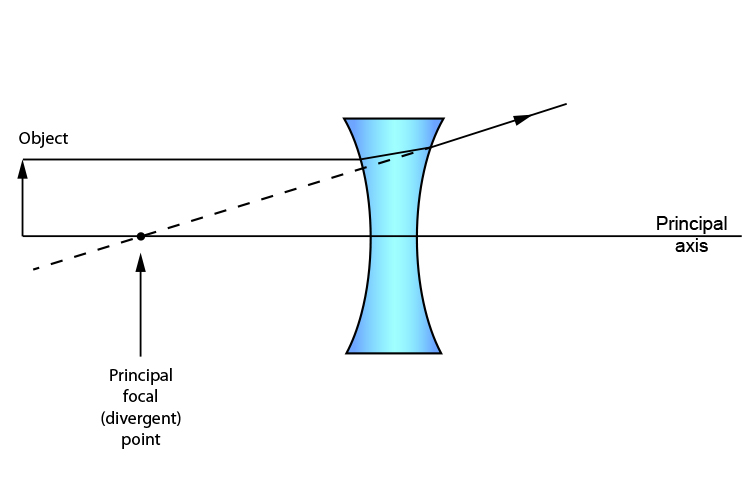1. From the object pass a ray line through the centre of the convex lens.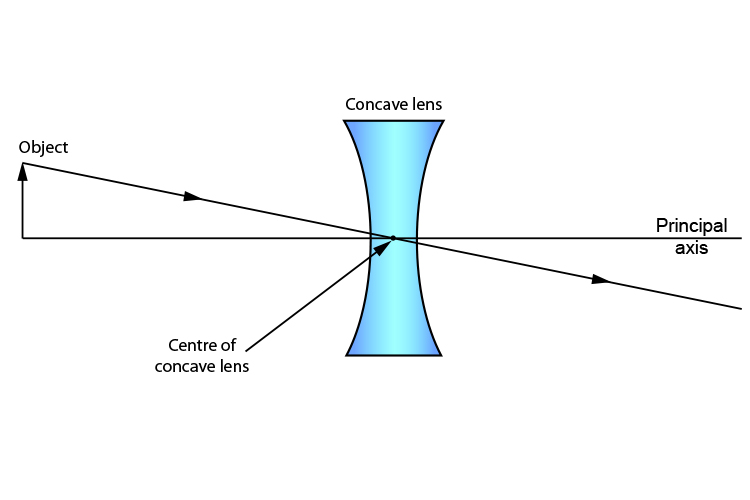Where the two lines intersect is the top of the object. Drop a line vertically down to the principal axis and you have your complete image.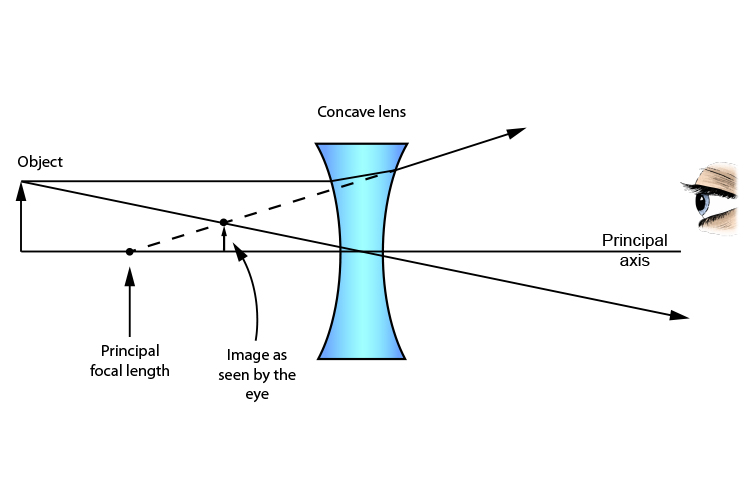And that is it. It’s that easy.

We could finish the lesson there but Mammoth Memory want to show you that you could in fact apply the same principal at any point on the object and the same ray diagram would work.

Lets try a ray diagram from the lower middle of the object.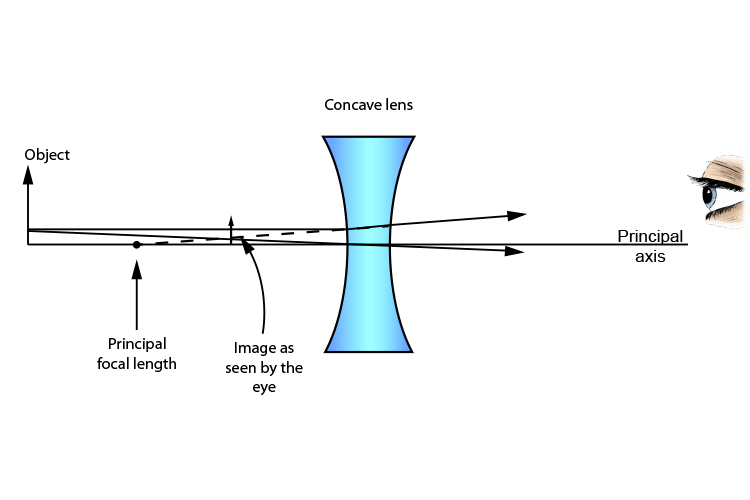As you can see, we can carry out this ray diagram method anywhere on the object, but again we emphasise that this is normally carried out from the top of the object.

Please note: Some books inform you that there is one more rule for drawing ray diagrams and that is: from the object, draw a line so that it travels towards the principal focal point on the opposite side of the lens. This ray will then refract through the concave lens and travel parallel to the principal axis. Extend the ray line behind the lens again parallel to the principal axis as follows: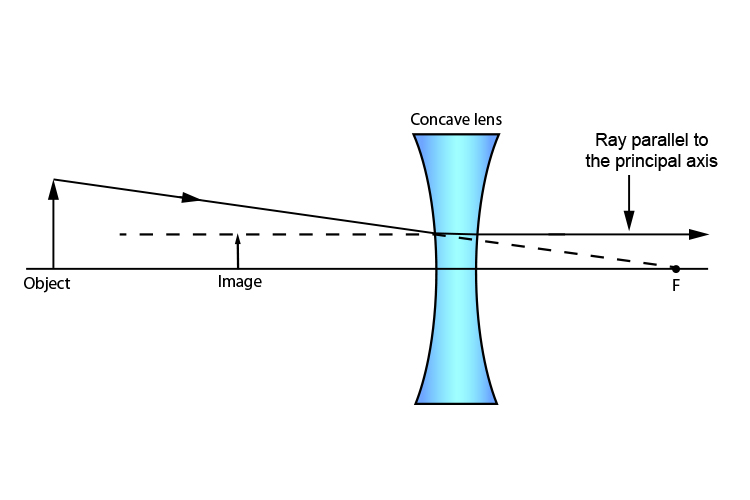Only the first two rules are needed.